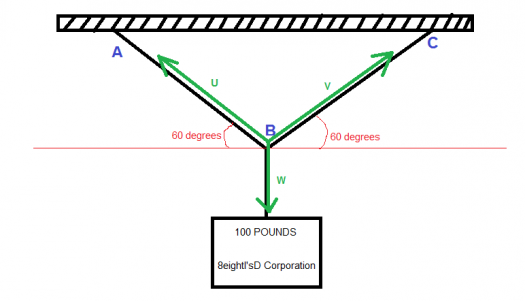# vector analysis

2 posts / 0 new
Benjie Magallano
vector analysis

how to solve the tension force of a two ropes connected in a box of 100lb and is being hang. the angles of the ropes are 60? Meaninig the box is in the center.

fitzmerl duronOn what is described in your problem, it would look like this:Upon closer understanding of the problem, it would look like this:To get the tension of the strings AB and BC, we can consider the string AB as vector $U$, the string BC as a vector $V$ and the resultant force
would be the weight of the box (symbolized as vector $W$)

So...

$$U+V=W$$

Then we set the point B as the origin of the rectangular coordinate system, so the new equation would be:

$$[X_1, Y_1] + [X_2, Y_2] = [X_3, Y_3]$$ $$[r_1\cos 60^o, r_1 \sin 60^o]+[r_2\cos 60^o, r_2\sin 60^o]=[100\cos 270^o, 100\sin 270^o]$$ $$[r_1\cos 60^o, r_1 \sin 60^o]+[r_2\cos 60^o, r_2\sin 60^o]=[0, -100]$$

Notice that the box was hanged on the middle of the string AC, so the tension in the strings AB and BC would be the same, so $U = V$

$$U+V=W$$ $$V+V=W$$ $$2V=W$$ $$2[r_2\cos 60^o, r_2\sin 60^o]=[0, -100]$$ $$[2r_2\cos 60^o, 2r_2\sin 60^o]=[0, -100]$$

Then doing this now:

$$2r_2\cos 60^o = 0$$ $$2r_2\sin 60^o = -100$$

Now getting the $r_2$ in $2r_2\cos 60^o = 0$:

$$r_2 = 0$$

That's not right....

Now getting the $r_2$ in $2r_2\sin 60^o = -100$:
$$r_2 = 57.7$$

We now conclude that the tension in one of the strings would be $57.7$ pounds.
To elaborate...tension in string AB would be $57.7$ pounds and the tension in the string BC would be $57.7$ pounds too...

Alternate solutions are encouraged:-)

• Mathematics inside the configured delimiters is rendered by MathJax. The default math delimiters are $$...$$ and $...$ for displayed mathematics, and $...$ and $...$ for in-line mathematics.